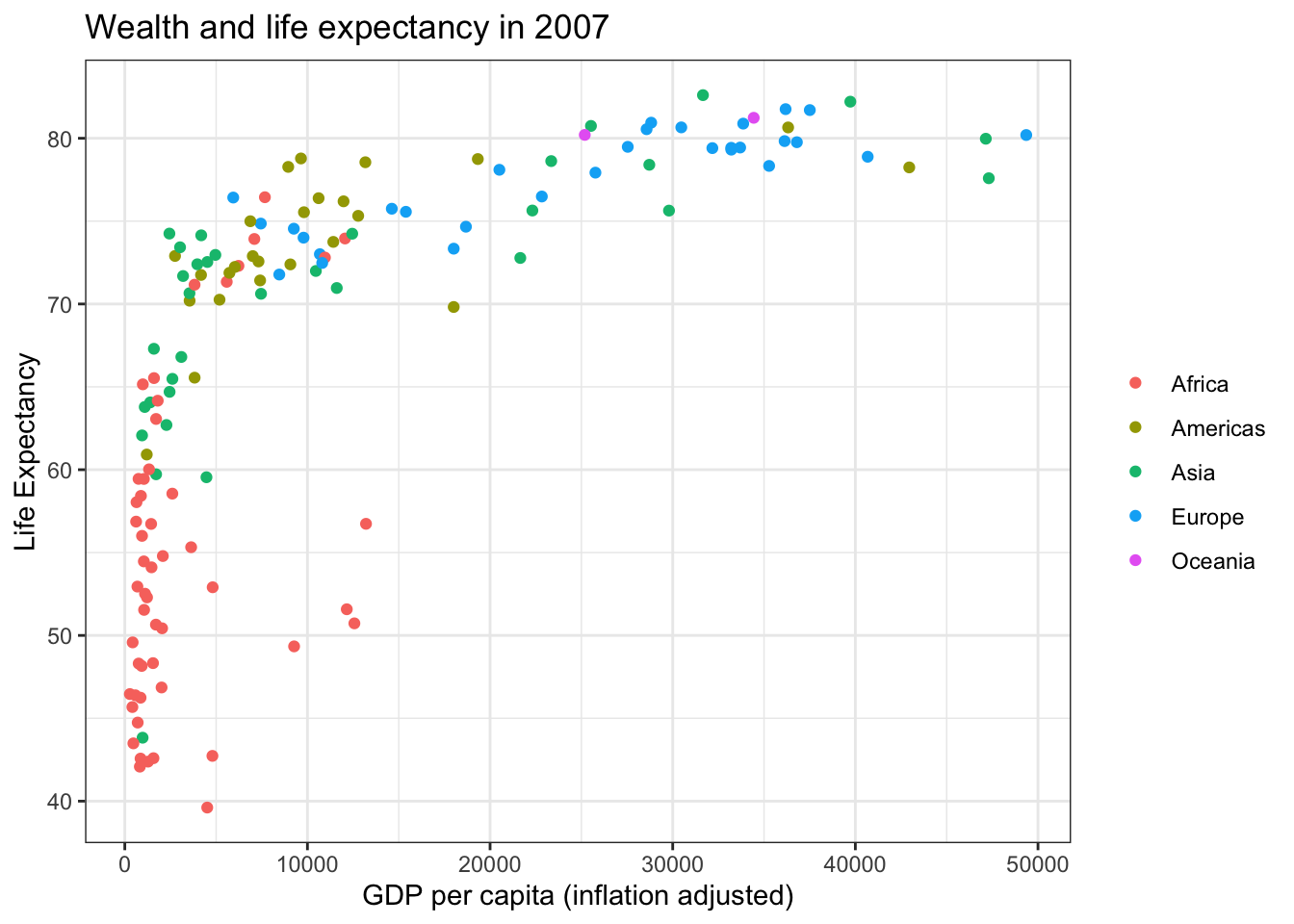# Slow ggplot

This post has lots in common with previous posts on “the layered presentation of graphics”. It is about building up plots, but now with a focus on this incremental change for teaching ggplot2. The rational is that observing the cause and effect of incremental change is easier to digest, and that the repetition in this approach means students have more chances to learn the ggplot2 functions.

My recent tweet presented the technique:

People reacted positively. Now here is the comparison between a traditional ggplot construction and the more verbose, slow ggplot construction.

``````library(tidyverse)
library(gapminder)
df_2007 <- gapminder %>% filter(year == 2007)``````

``````ggplot(data = df_2007,
mapping = aes(x = gdpPercap,
y = lifeExp,
col = continent)) +
geom_point() +
labs(title = "Wealth and life expectancy in 2007",
x = "GDP per capita (inflation adjusted)",
y = "Life Expectancy",
col = "") +
theme_bw()``````Verbose, slow approach:

``````ggplot(data = df_2007) +
aes(x = gdpPercap) +
aes(y = lifeExp) +
geom_point() +
aes(col = continent) +
labs(title = "Wealth and life expectancy in 2007") +
labs(x = "GDP per capita (inflation adjusted)") +
labs(y = "Life Expectancy") +
labs(col = "") +
theme_bw()``````Same result, but students are perhaps more likely to go slow, and mentally make connections between adjustment to product and the functions for making those adjustments, given that each change gets its own line of code and that there is repetition built into this exercise. After using this approach to introduce students to ggplot, students can be alerted to the fact that they can gather up arguments into one function.

# Downsides?

A possible downside is that most teaching materials don’t take this approach. Perhaps it will be confusing to have the two approaches in students’ head. This is an unanswered empirical question, but my hunch is that newbies won’t find this jump so hard to make.

##### Evangeline Reynolds
###### Visiting Teaching Assistant Professor

My research interests include international institutions, causal inference, data visualization, and computational social science and pedagogy.